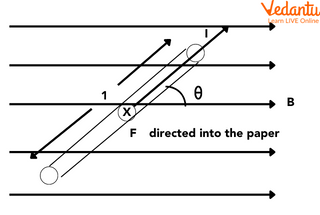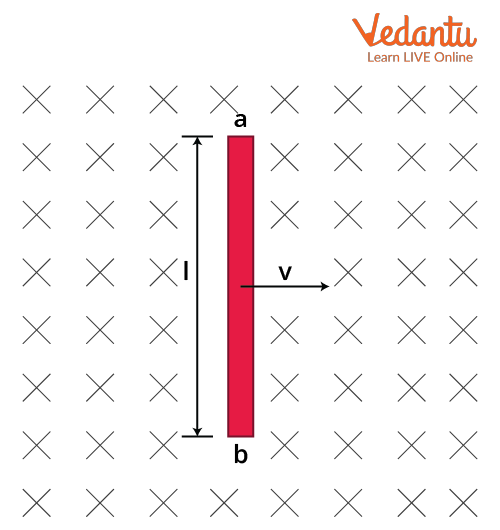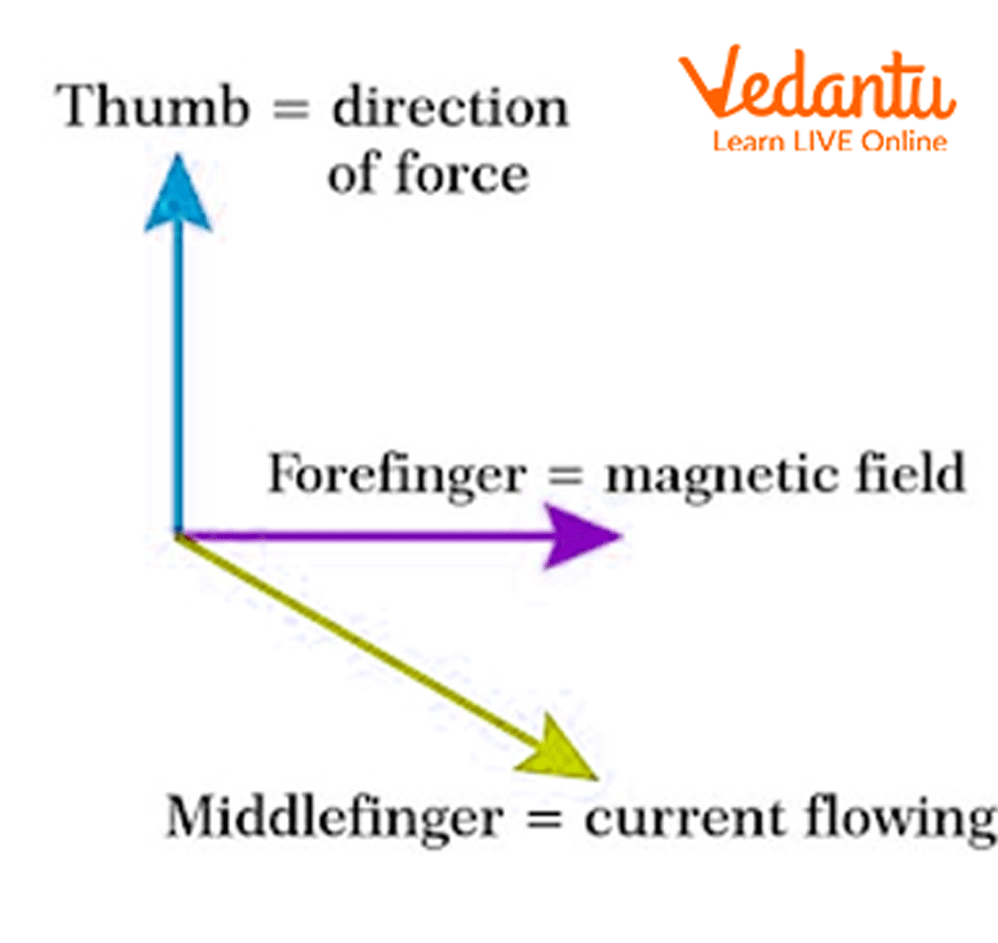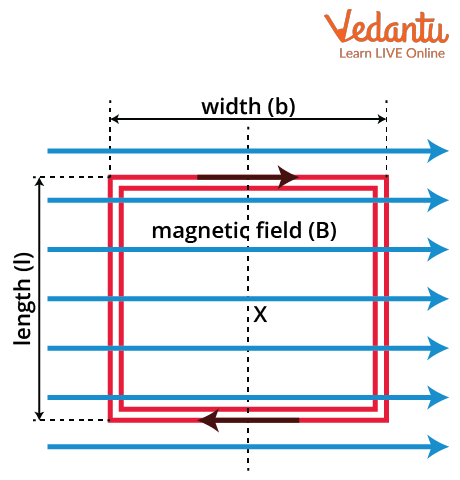Courses
Courses for Kids
Free study material
Free LIVE classes
More

# Force on a Current Carrying Conductor in a Magnetic Field for JEE Exam## Force on a Current-carrying Conductor and Fleming's Left-hand Rule

Last updated date: 20th Mar 2023
Total views: 77.4k
Views today: 0.55k

Electric and magnetic fields both have their own significance. As we know, electricity is produced by a varying magnetic field. Also, when we place a current-carrying conductor in a magnetic field, it experiences a force. The direction of this force is given by Fleming’s left-hand rule, which will be thoroughly discussed in this article. Additionally, the force on a current-carrying conductor in a magnetic field is given by the vector product magnetic field density and the differential length of the conductor or $F=i(dl\times B)$.

## Magnetic Force on Current-carrying Conductor

As discussed earlier, when a current-carrying conductor is placed in a magnetic field, it experiences a force. The magnitude of this force is given by $F=i(dl\times B)$----(1)

or $F=(BIl)$-----(3)

The above equation can be further expanded as $F=B I l \sin (\theta)$-----(4)Force on a Current-Carrying ConductorForce on a Moving Conductor in a Magnetic Field

Similarly, when a conducting conductor is moved through a magnetic field with a velocity ‘v’, every charge on the conductor experiences a force. The magnitude of this force is denoted as $F=q(v\times B)$----(2)

There is also a special case when the force acting on a current conductor is zero. In the case of a closed-loop equation (3) becomes $F=i(0\times B)=0$.

## How to Know the Direction of Force on a Current-carrying Conductor?

We have discussed the concept of force acting on a current-carrying conductor in a magnetic field. Now, let us understand how to find the direction of this force.

## Fleming’s Left Hand Rule

Fleming’s left-hand rule definition states that if we stretch the thumb, middle finger and the forefinger of our left-hand, perpendicular to one another, then the middle finger denotes the direction of the magnetic field, the forefinger denotes the current and the thumb denotes the direction of force in the magnetic field.Fleming’s Left-hand Rule

### Fleming’s Left-hand Rule Applications

One of the best examples of using Fleming’s left-hand rule is in the case of motors. In a motor, both current and magnetic fields exist. The interaction of the electromagnetic and magnetic fields creates a force. In the case of a motor, the direction of this force is found out by Fleming’s left-hand rule. The article elaborates the use of Fleming's left-hand rule with some examples. Additionally, a few examples that calculate the force on a conductor have also been given above.

## Examples of a Magnetic Force Acting on a Conductor

Example 1: A wire of length 0.6 m carrying a current of 2 A is placed at right angles to a magnetic field of 0.3 T. Calculate the force on the wire.

Solution:

The force on a current-carrying conductor is denoted as $F=Bil\sin{\theta}$ and we know that   $\theta=90^{\circ}$

$F=2\times 0.6\times0.3$

F = 0.36 N.

So the force on the wire is 0.36N.

Example 2: A force of 0.25 N is exerted by a magnetic field on a wire of 8 cm length, which is carrying a current of 3 Ampere placed at right angles to the field. Calculate the force on a 30 cm long wire with the same field and current.

Solution:

To calculate the force for a 30 cm length of wire,

\begin{align} &F=0.25 \times \dfrac{30}{8} \\ &F=0.937 \mathrm{~N} \end{align}

The above relation is deduced as force is proportional to the length from equation (3).

The force on the wire is 0.937N.

Example 3: Calculate:

(a) Calculate the angle between a current-carrying wire of 6 A and the 1.3 T magnetic field it is placed in, if a 40 cm length of wire experiences a magnetic force of 3.40 N.

(b) Calculate the force on the wire when it is rotated to make an angle of 900 with the field.

Solution:

From equation (4), $F=BIl\sin{(\theta)}$, we have

\begin{align} &\sin (\theta)=\dfrac{F}{B I l} \\ &\sin (\theta)=\dfrac{3.40}{1.3 \times 6 \times 40} \\ &\theta=0.623 \end{align}

(b) From equation (4), $F=BIl\sin{(\theta)}$ we have,

\begin{align} &F=1.3 \times 6 \times 40 \times \sin \left(90^{\circ}\right) \\ &F=312 N \end{align}

Example 4:  A straight conductor carries a current of 5 A. An electron travelling with a speed of $5\times10^6~\dfrac{m}{s}$ parallel to the wire at a distance of 0.1m from the conductor experiences a force of

Solution:

The magnetic field produced by a wire is given as

$B=\dfrac{\mu_{0}}{4 \pi} \dfrac{2 i}{a}$

i=5A and a=0.1m.

So the magnetic field produced by the wire will be

\begin{align} &B=10^{-7} \times \dfrac{2 \times 5}{0.1} \\ &B=10^{-5} ~\dfrac{\mathrm{Wb}}{ \mathrm{m}^{2}} \end{align}

The force on the electron can be given by lorentz force formula which is,

F=qvB

We have $v=5\times10^6~\dfrac{m}{s}$.

So the force will be,

\begin{align} &F=\left(1.6 \cdot 10^{-19}\right) \times 5 \times 10^{6} \times 10^{-5} \\ &F=8 \times 10^{-18} \mathrm{~N} \end{align}

So the force acting on the electron will be 8$\times$10-18 N.

## Conclusion

The article presents a thorough understanding of the current-carrying conductor in a magnetic field or when the conductor is in motion in the field. Fleming’s left-hand rule plays an important role here, as it denotes the direction of the force in a current-carrying conductor placed in a magnetic field. The above concept is thoroughly explained using different examples as well.

## FAQs on Force on a Current Carrying Conductor in a Magnetic Field for JEE Exam

1. What is the measure of force between two parallel current-carrying conductors?

Two parallel conductors placed in a magnetic field exert a force on one another. The magnetic field produced by both of them interacts with one another to develop a force between the two conductors. This force may be repulsive or attractive, depending on the direction of current in these conductors. The force acting between two parallel current-carrying conductors is denoted as $F=\dfrac{\mu_{o} I_{1} I_{2}}{2 \pi r}$. Here, I1 and I2 are the currents in conductor 1 and conductor 2, respectively, and r is the distance between the two conductors.

2. What is the torque acting on a current-carrying coil?The figure above denotes a current-carrying coil placed in a magnetic field. The torque acting on this coil is calculated as

\begin{align} &\zeta=b \times F \\ &\zeta=B I l \sin (\theta) \times b \\ &\zeta=B I \sin (\theta)(l \times b) \\ &\zeta=B I \sin (\theta) A \\ &\zeta=B I A \sin (\theta) \end{align}

For a coil with n turns, the torque produced will be: $\zeta=n B I A \sin (\theta)$

If the plane of the coil makes an angle $\beta$, then the torque is denoted as

Further, if the plane of the coil is perpendicular to $\vec \beta$, the magnetic field then torque $\zeta=0$

If the plane of the coil is parallel to $\vec \beta$, then torque $\zeta=BINA$